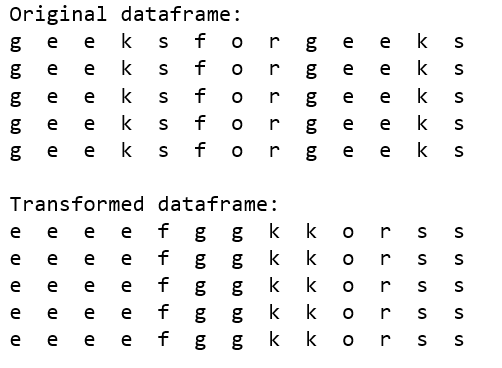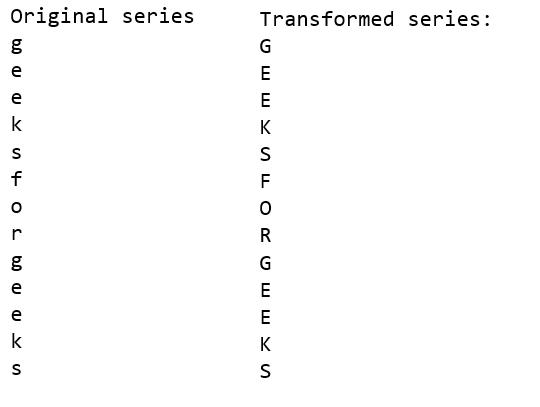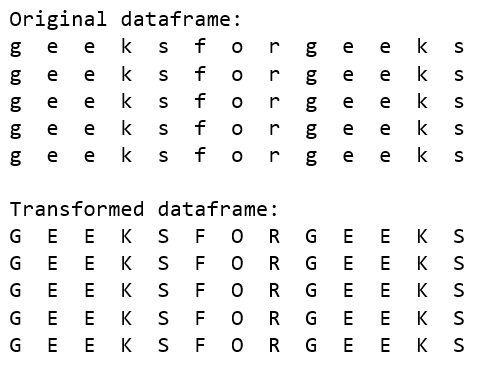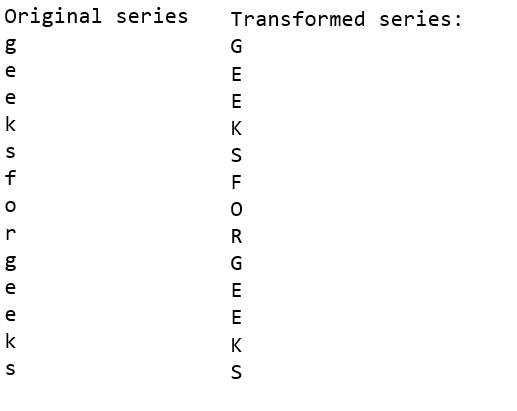# Difference between map, applymap and apply methods in Pandas

Pandas library is extensively used for data manipulation and analysis. `map()`, `applymap()` and `apply()` methods are methods of Pandas library.

`applymap()` method only works on a pandas dataframe where function is applied on every element individually.

`apply()` method can be applied both to series and dataframes where function can be applied both series and individual elements based on the type of function provided.

`map()` method only works on a pandas series where type of operation to be applied depends on argument passed as a function, dictionary or a list.

Note that the type of Output totally depends on the type of function used as an argument with the given method.

Pandas `apply()` method:
This method which can be used on both on a pandas dataframe and series. The function passed as an argument typically works on rows/columns. Code below illustrates how `apply()` method works on Pandas dataframe.

 `# Importing pandas library with an alias pd ` `import` `pandas as pd ` `  `  `# Dataframe generation ` `gfg_string ``=` `'geeksforgeeks'` `gfg_list ``=` `5` `*` `[pd.Series(``list``(gfg_string))] ` `  `  `gfg_df ``=` `pd.DataFrame(data ``=` `gfg_list) ` `print``(``"Original dataframe:\n"` `+` `\ ` `      ``gfg_df.to_string(index ``=` `False``, ` `      ``header ``=` `False``), end ``=` `'\n\n'``) ` `  `  `# Using apply method for sorting  ` `# rows of characters present in  ` `# the original dataframe ` `new_gfg_df ``=` `gfg_df.``apply``(``lambda` `x:x.sort_values(), axis ``=` `1``) ` ` `  `print``(``"Transformed dataframe:\n"` `+` `\ ` `       ``new_gfg_df.to_string(index ``=` `False``, ` `            ``header ``=` `False``), end ``=` `'\n\n'``) `

Output:Below Code illustrates how `apply()` method on Pandas series:

 `# Importing pandas library with an alias pd ` `import` `pandas as pd ` `  `  `# Series generation ` `gfg_string ``=` `'geeksforgeeks'` `gfg_series ``=` `pd.Series(``list``(gfg_string)) ` `print``(``"Original series\n"` `+` `\ ` `       ``gfg_series.to_string(index ``=` `False``, ` `            ``header ``=` `False``), end ``=` `'\n\n'``) ` `  `  `# Using apply method for converting characters ` `# present in the original series ` `new_gfg_series ``=` `gfg_series.``apply``(``str``.upper) ` `print``(``"Transformed series:\n"` `+` `\ ` `       ``new_gfg_series.to_string(index ``=` `False``, ` `                ``header ``=` `False``), end ``=` `'\n\n'``) `

Output:Pandas `applymap()` method :
This method can be used on a pandas dataframe. The function passed as an argument typically works on elements of the dataframe `applymap()` is typically used for elementwise operations. Code below illustrates how `applymap` method works on pandas dataframe:

 `# Importing pandas library with an alias pd ` `import` `pandas as pd ` `  `  `# DataFrame generation ` `gfg_string ``=` `'geeksforgeeks'` `gfg_list ``=` `5` `*` `[pd.Series(``list``(gfg_string))] ` `gfg_df ``=` `pd.DataFrame(data ``=` `gfg_list) ` ` `  `print``(``"Original dataframe:\n"` `+` `\ ` `       ``gfg_df.to_string(index ``=` `False``, ` `        ``header ``=` `False``), end ``=` `'\n\n'``) ` `  `  `# Using applymap method for transforming  ` `# characters into uppercase characters  ` `# present in the original dataframe ` `new_gfg_df ``=` `gfg_df.applymap(``str``.upper) ` `print``(``"Transformed dataframe:\n"` `+` `\ ` `       ``new_gfg_df.to_string(index ``=` `False``, ` `            ``header ``=` `False``), end ``=` `'\n\n'``) `

Output:Pandas `map()` method :
This method is used on series function, list and dictionary passed as an argument. This method is generally used to map values from two series having one column same. Code below illustrates how `map` method works on pandas series:

 `# Importing pandas library with an alias pd ` `import` `pandas as pd ` `  `  `# Series generation ` `gfg_string ``=` `'geeksforgeeks'` `gfg_series ``=` `pd.Series(``list``(gfg_string)) ` `print``(``"Original series\n"` `+` `\ ` `       ``gfg_series.to_string(index ``=` `False``, ` `            ``header ``=` `False``), end ``=` `'\n\n'``) ` `  `  `# Using apply method for converting characters ` `# present in the original series ` `new_gfg_series ``=` `gfg_series.``map``(``str``.upper) ` `print``(``"Transformed series:\n"` `+` `\ ` `       ``new_gfg_series.to_string(index ``=` `False``, ` `                ``header ``=` `False``), end ``=` `'\n\n'``) `

Output:My Personal Notes arrow_drop_upCheck out this Author's contributed articles.

If you like GeeksforGeeks and would like to contribute, you can also write an article using contribute.geeksforgeeks.org or mail your article to contribute@geeksforgeeks.org. See your article appearing on the GeeksforGeeks main page and help other Geeks.

Please Improve this article if you find anything incorrect by clicking on the "Improve Article" button below.

Article Tags :

2

Please write to us at contribute@geeksforgeeks.org to report any issue with the above content.